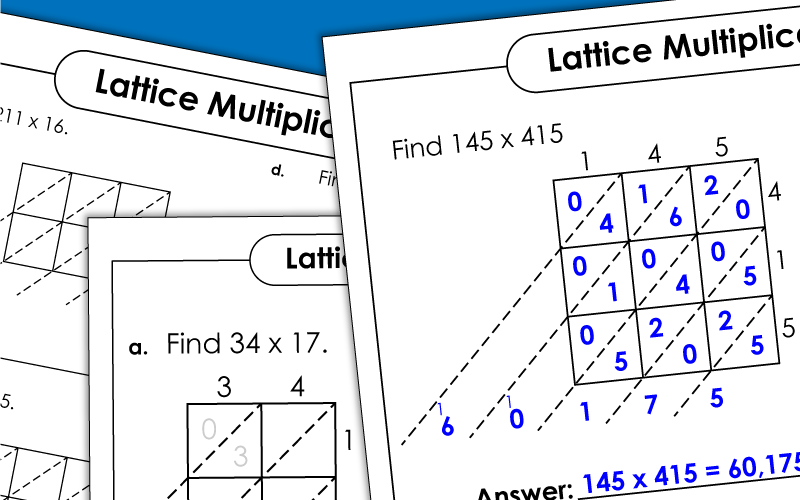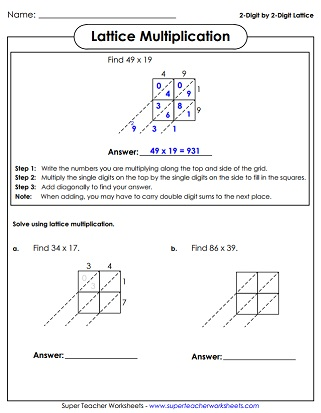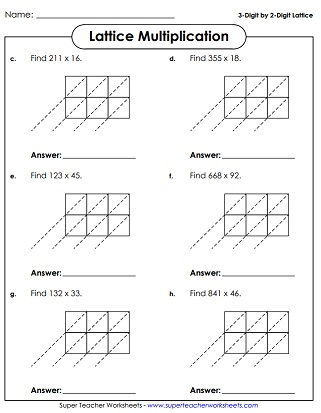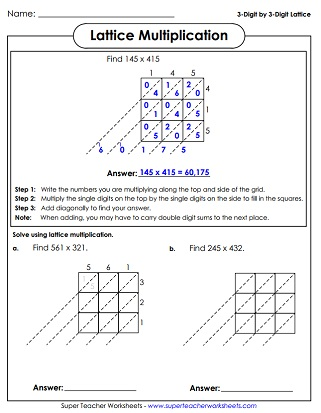# Lattice Multiplication Worksheets

Lattice multiplication is a fun way of solving long multiplication problems with a grid.  This page contains lattice grids of various sizes.  There are also several practice worksheets that explain how to use the lattice method to solve multiplication problems.## 2-Digit by 2-Digit

Worksheet gives an example and explains the steps to solving lattice multiplication problems.  There are also several practice problems for students to try.
Print out a page of blank lattice multiplication grids to help your students solve problems quickly and accurately.

## 3-Digit By 2-Digit

Shows the steps to solving lattice multiplication problems and gives an example, followed by many practice problems for students to try on their own.
A pages with lots of blank 3-by-2 grids to print out.

## 3-Digit by 3-Digit

Once your students have mastered simpler lattice multiplication grids, have them try these large 3-digit by 3-digit problems.

## Related Page on SuperTeacherWorksheets

Multiplication Basic Facts

Tons of multiplication games, activities, and practice worksheets

## Sample Worksheet ImagesMy Account
Site Information